JAJSEU1B November   2017  – May 2018

PRODUCTION DATA.

1. 特長
2. アプリケーション
3. 概要
1.     Device Images
4. 改訂履歴
5. Device Comparison Table
6. Pin Configuration and Functions
7. Specifications
8. Detailed Description
1. 8.1 Overview
2. 8.2 Functional Block Diagram
3. 8.3 Feature Description
4. 8.4 Device Functional Modes
9. Application and Implementation
1. 9.1 Application Information
2. 9.2 Typical Application
10. 10Power Supply Recommendations
11. 11Layout
12. 12デバイスおよびドキュメントのサポート
13. 13メカニカル、パッケージ、および注文情報

• DLC|8
• DLC|8

#### 9.2.3 Application Curves

VIN=5V, VOUT=1.8V, TA=25°C, BOM = Table 2, (unless otherwise noted)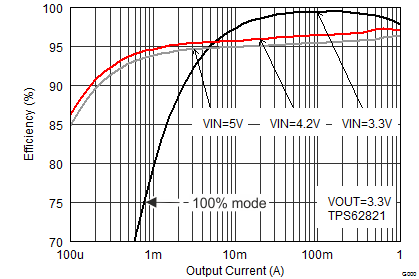Figure 8. Efficiency TPS62821 at VOUT=3.3V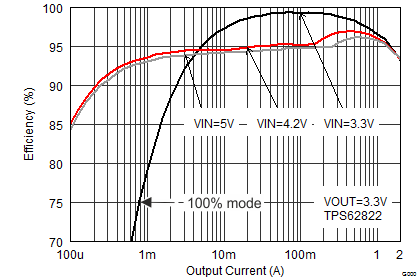Figure 10. Efficiency TPS62822 at VOUT=3.3VFigure 12. Efficiency TPS62823 at VOUT=3.3V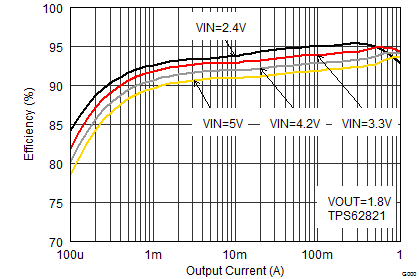Figure 14. Efficiency TPS62821 at VOUT=1.8V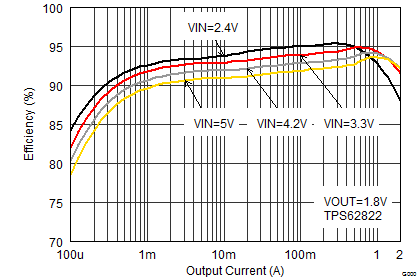Figure 16. Efficiency TPS62822 at VOUT=1.8V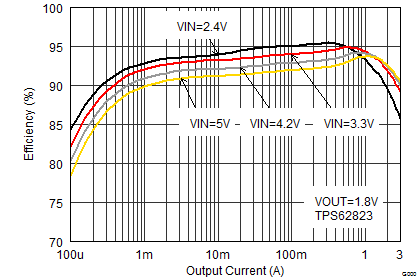Figure 18. Efficiency TPS62823 at VOUT=1.8V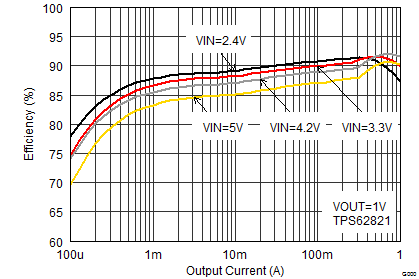Figure 20. Efficiency TPS62821 at VOUT=1V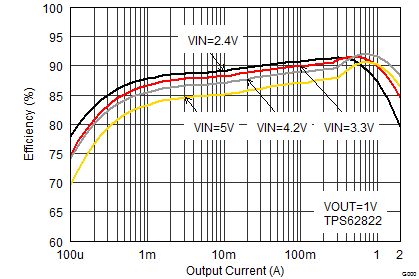Figure 22. Efficiency TPS62822 at VOUT=1V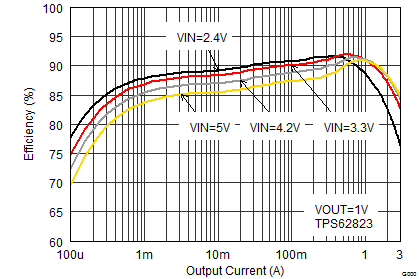Figure 24. Efficiency TPS62823 at VOUT=1V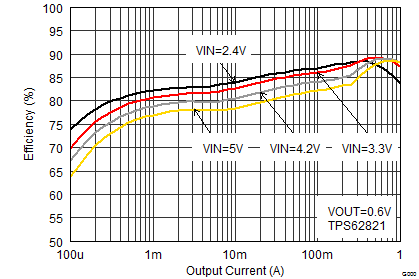Figure 26. Efficiency TPS62821 at VOUT=0.6V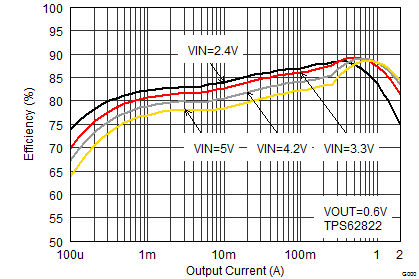Figure 28. Efficiency TPS62822 at VOUT=0.6V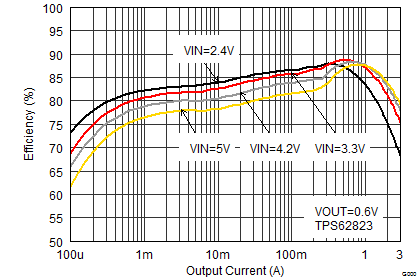Figure 30. Efficiency TPS62823 at VOUT=0.6V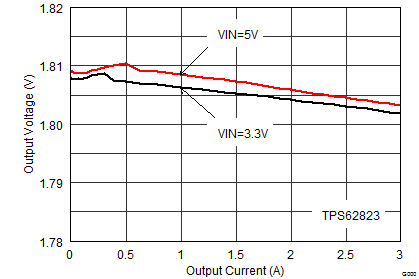Figure 32. Output Voltage Accuracy (Load Regulation)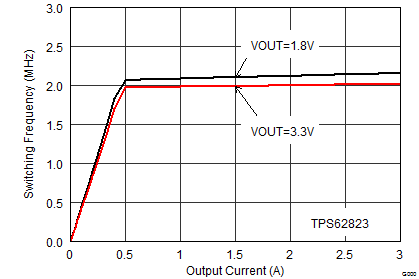Figure 34. Switching Frequency vs Output Current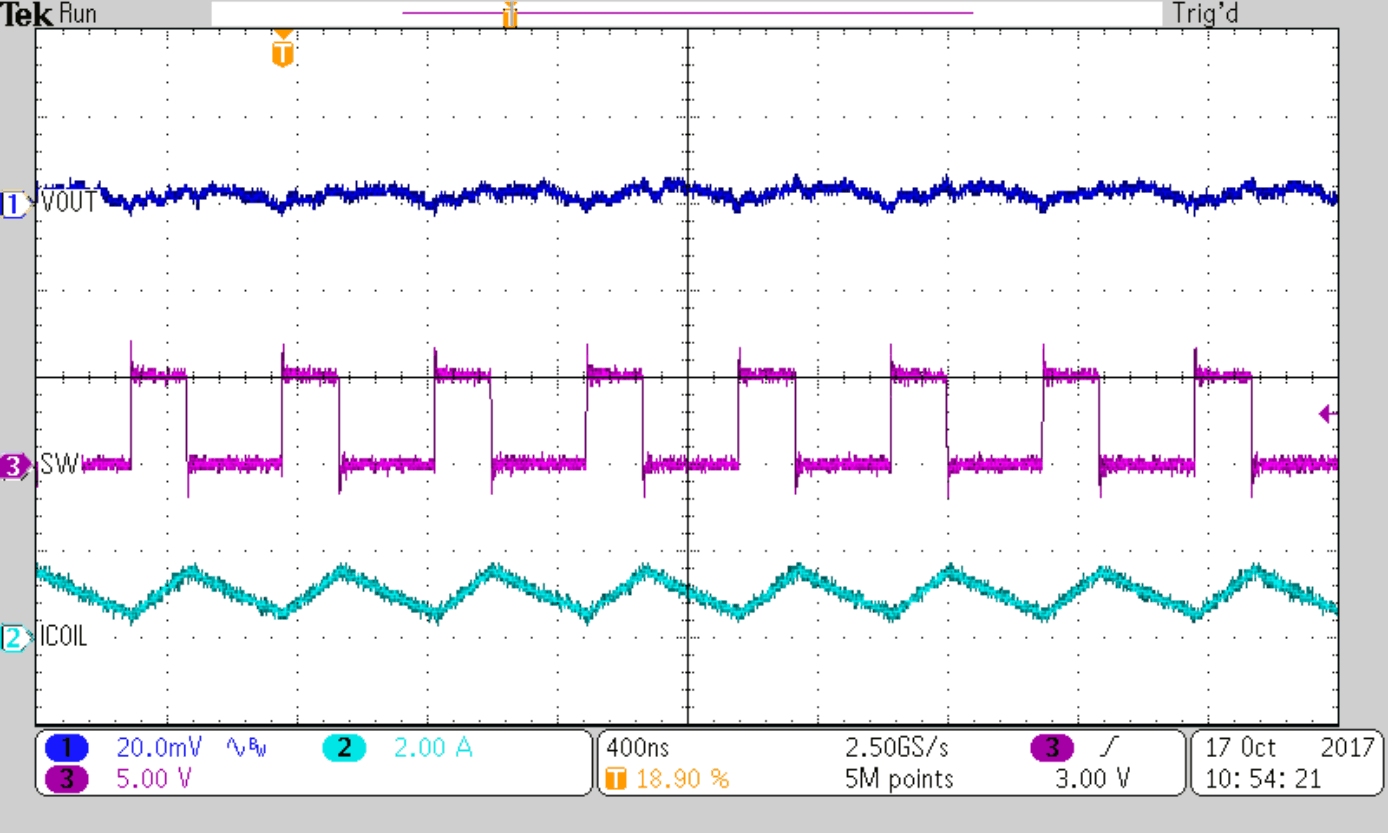COUT=2x10µF IOUT=1A
Figure 36. Typical Operation PWM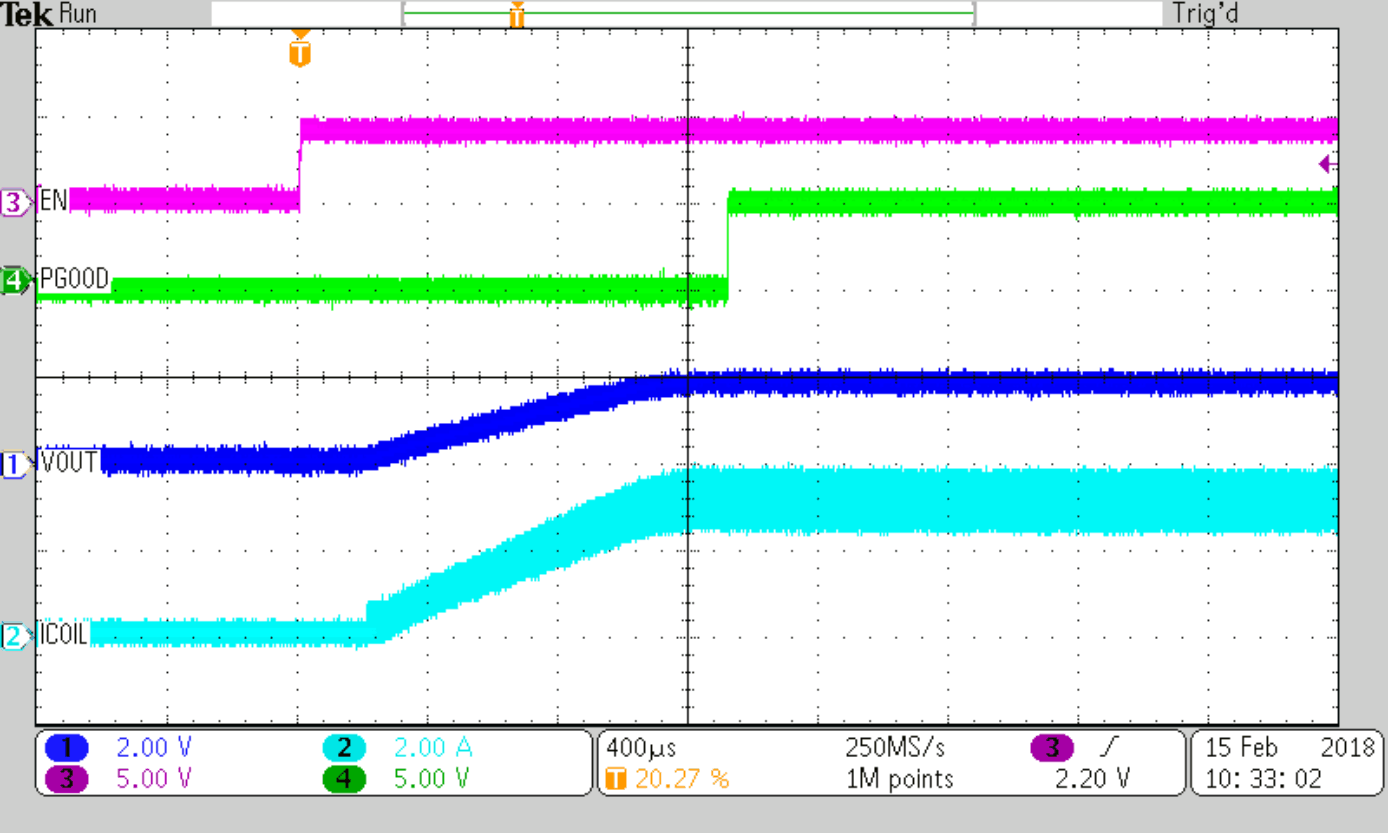COUT=2x10µF
Figure 38. Startup into 0.6-Ohm (TPS62823)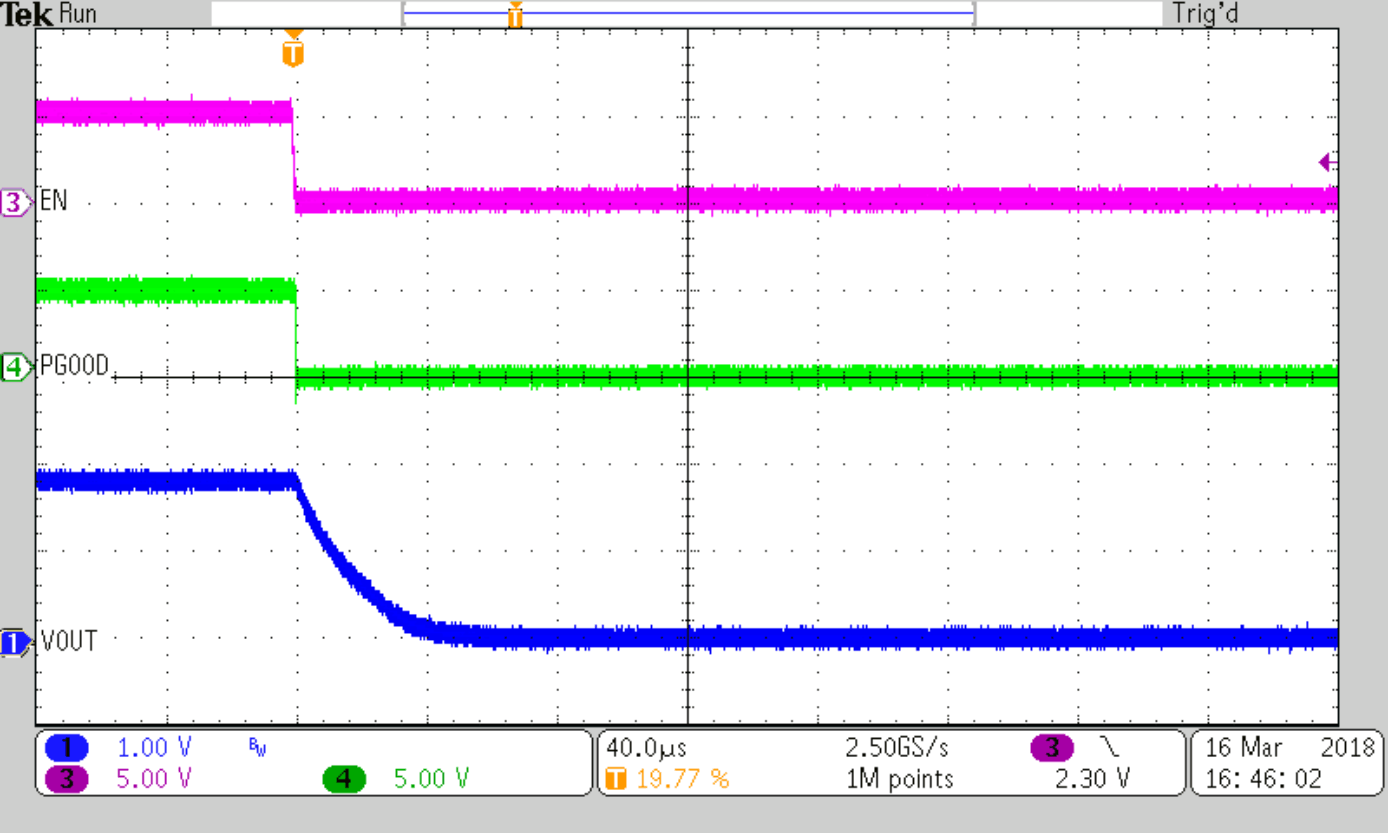COUT=2x10µF
Figure 40. Active Output Discharge at load 1.8-OhmCOUT=2x10µF Cff=120pF
Figure 42. Load Transient Response, 50mA to 1A, TPS62822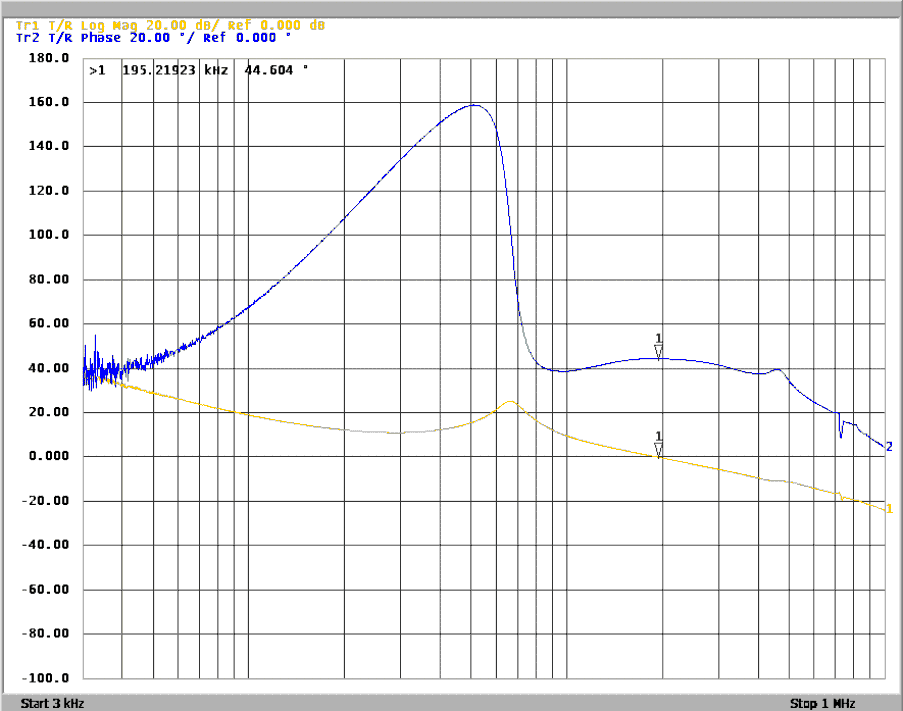COUT=2x10µF no CFF
Figure 44. Frequency Response (TPS62823), IOUT=3A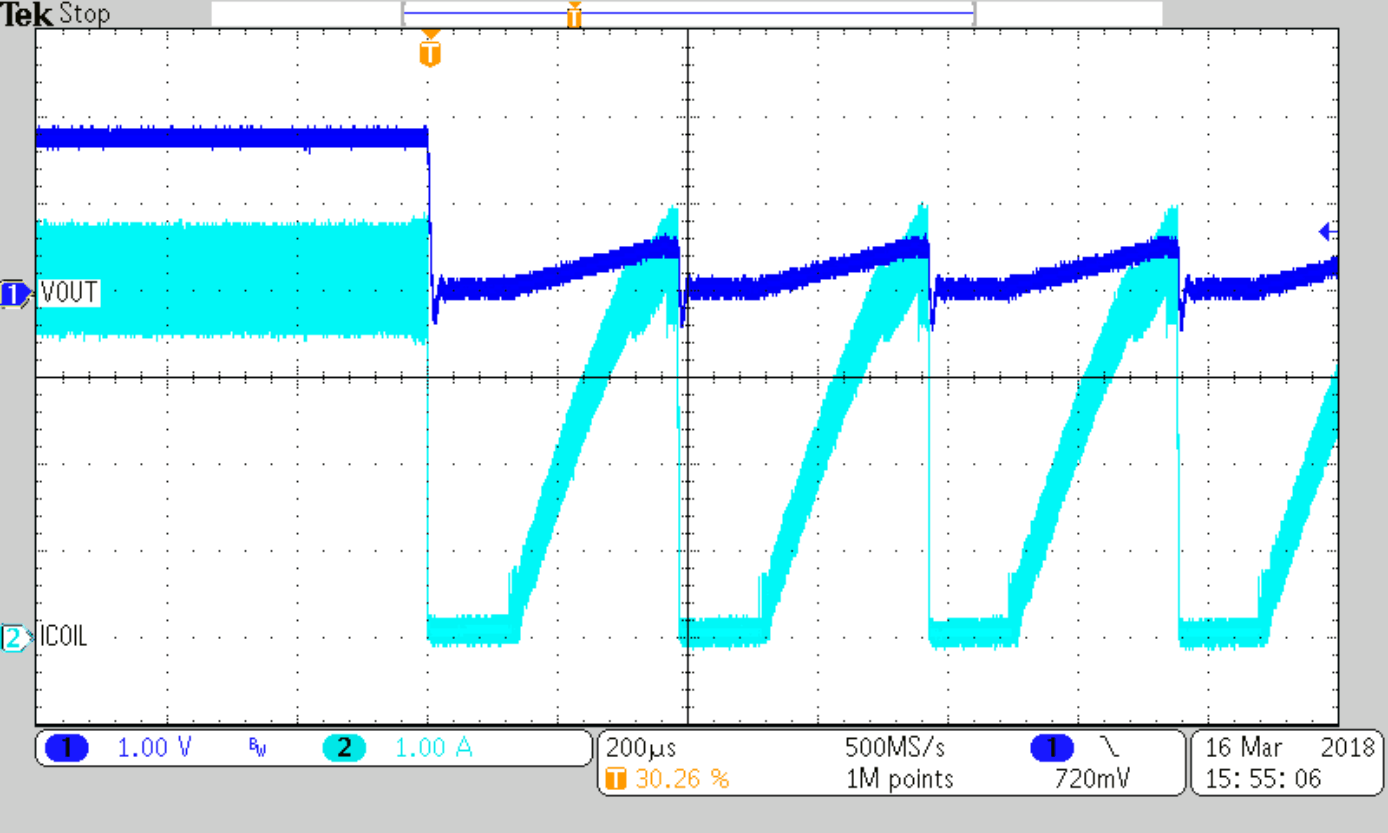Figure 46. Overload Response of TPS62823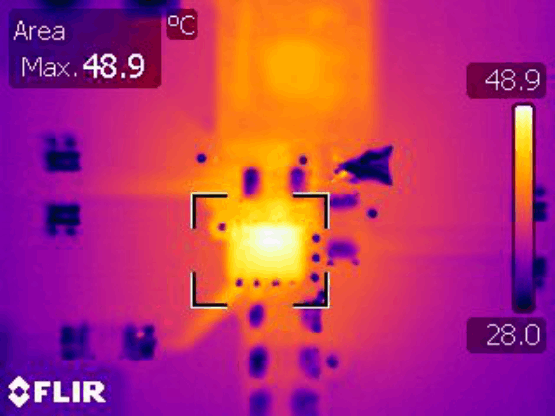Figure 48. Device Temperature Rise on TPS62823 EVM at IOUT=3A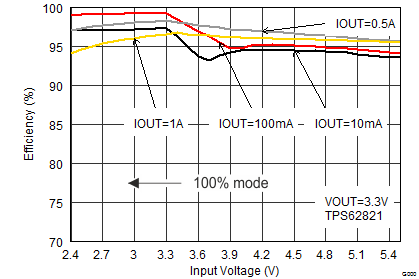Figure 9. Efficiency TPS62821 at VOUT=3.3V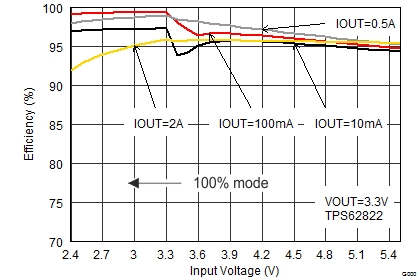Figure 11. Efficiency TPS62822 at VOUT=3.3V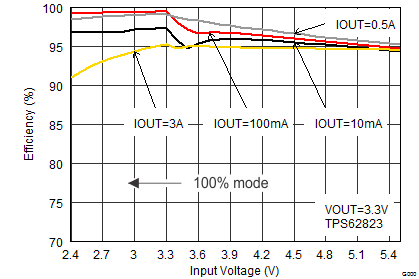Figure 13. Efficiency TPS62823 at VOUT=3.3V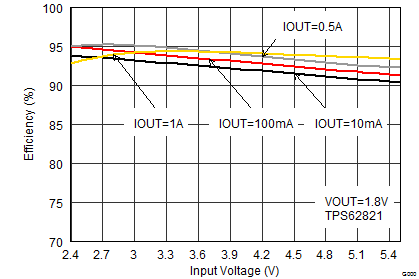Figure 15. Efficiency TPS62821 at VOUT=1.8V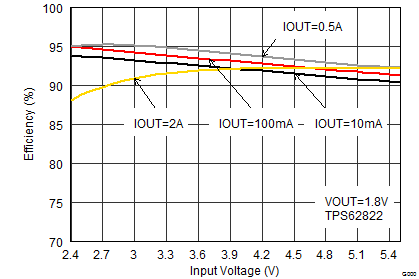Figure 17. Efficiency TPS62822 at VOUT=1.8V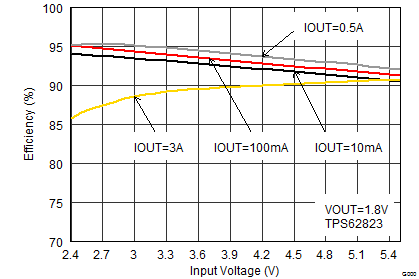Figure 19. Efficiency TPS62823 at VOUT=1.8V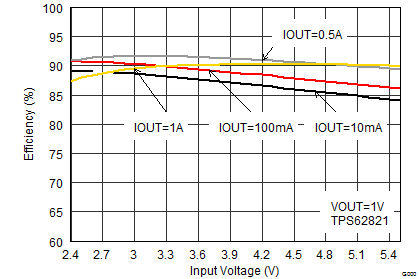Figure 21. Efficiency TPS62821 at VOUT=1VFigure 23. Efficiency TPS62822 at VOUT=1V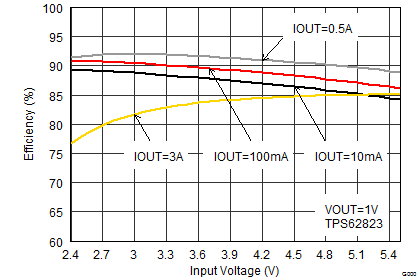Figure 25. Efficiency TPS62823 at VOUT=1V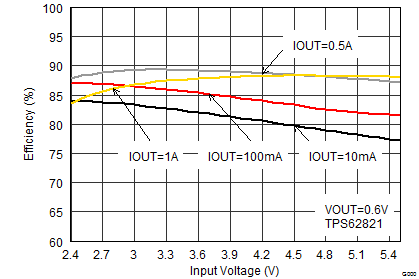Figure 27. Efficiency TPS62821 at VOUT=0.6V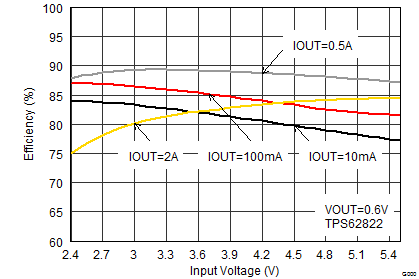Figure 29. Efficiency TPS62822 at VOUT=0.6V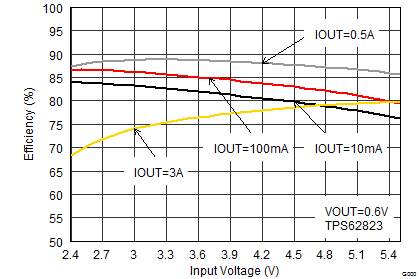Figure 31. Efficiency TPS62823 at VOUT=0.6V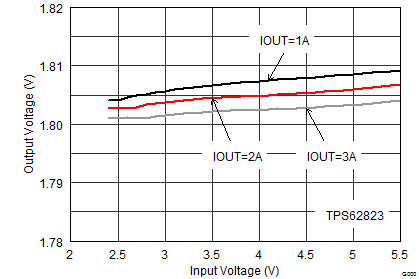Figure 33. Output Voltage Accuracy (Line Regulation)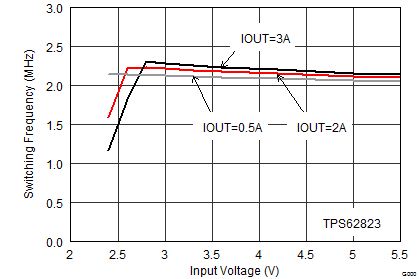Figure 35. Switching Frequency vs Input Voltage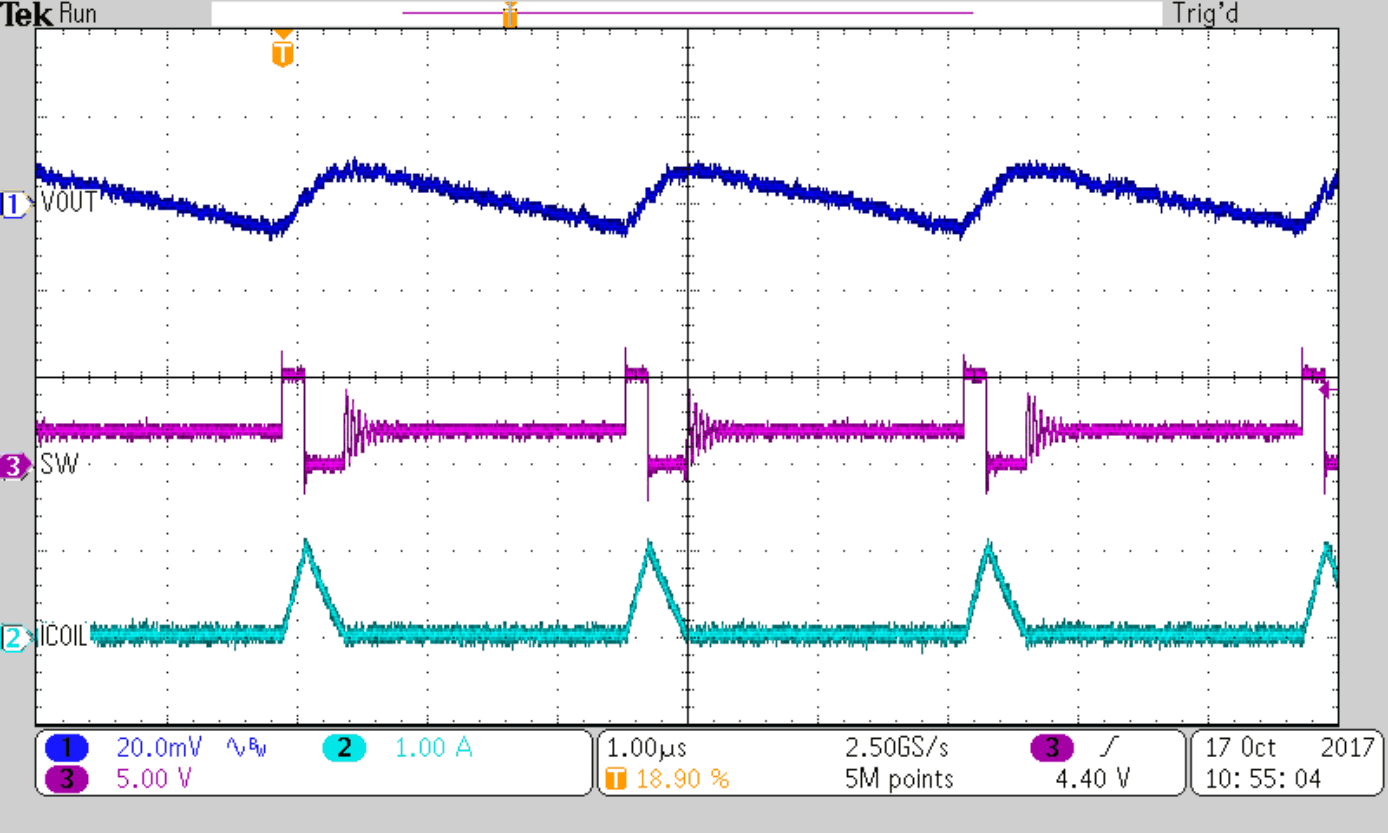COUT=2x10µF IOUT=0.1A
Figure 37. Typical Operation PSM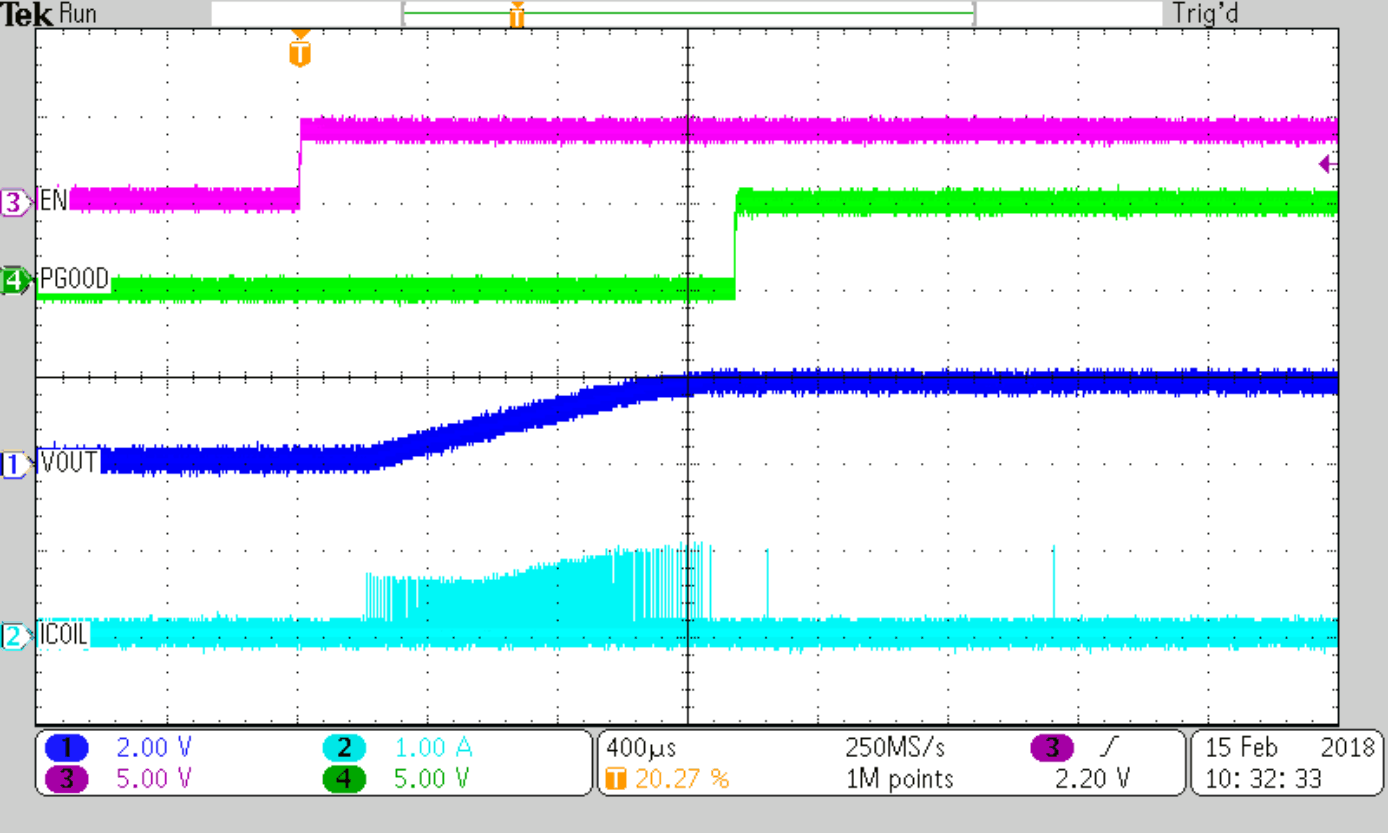COUT=2x10µF
Figure 39. Startup at No Load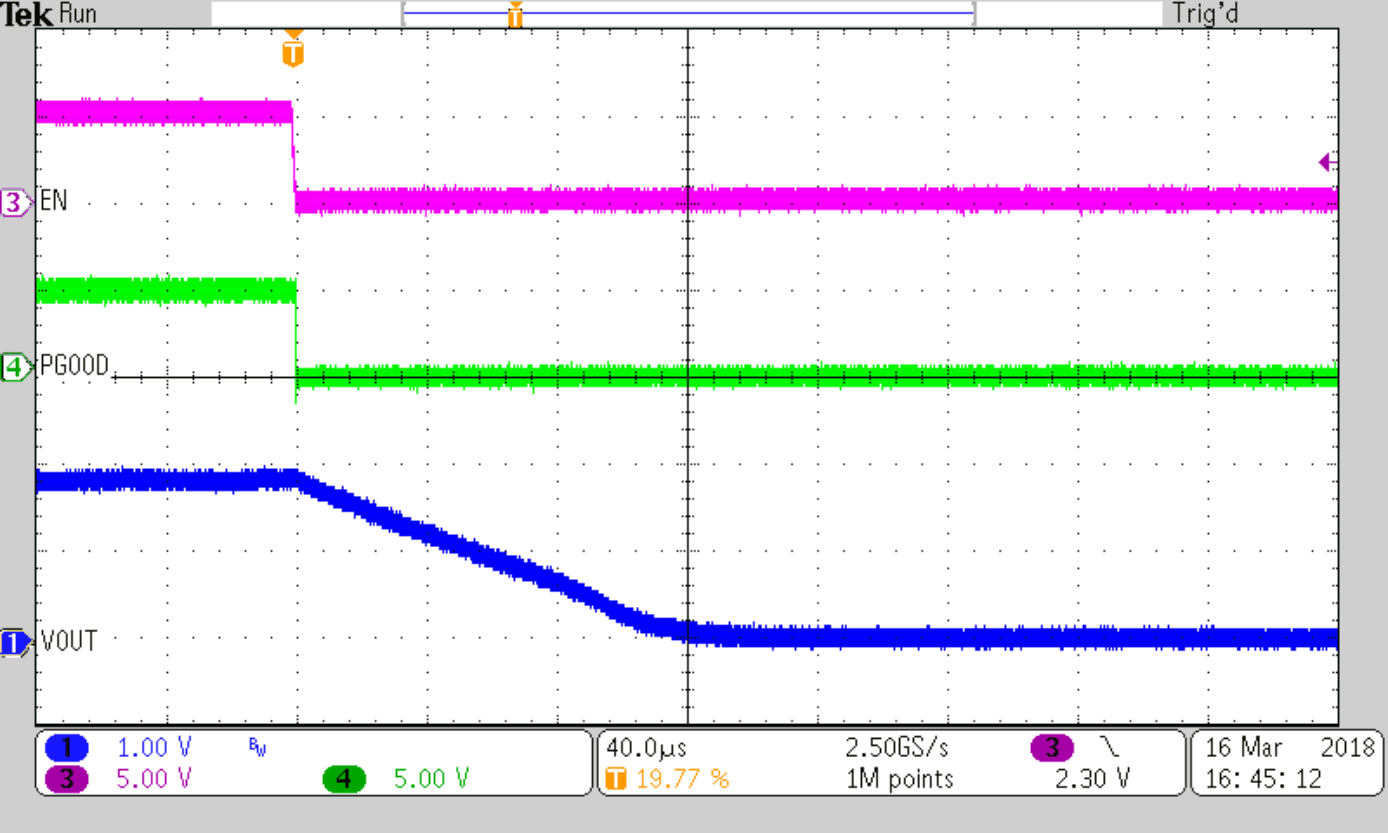COUT=2x10µF
Figure 41. Active Output Discharge at No Load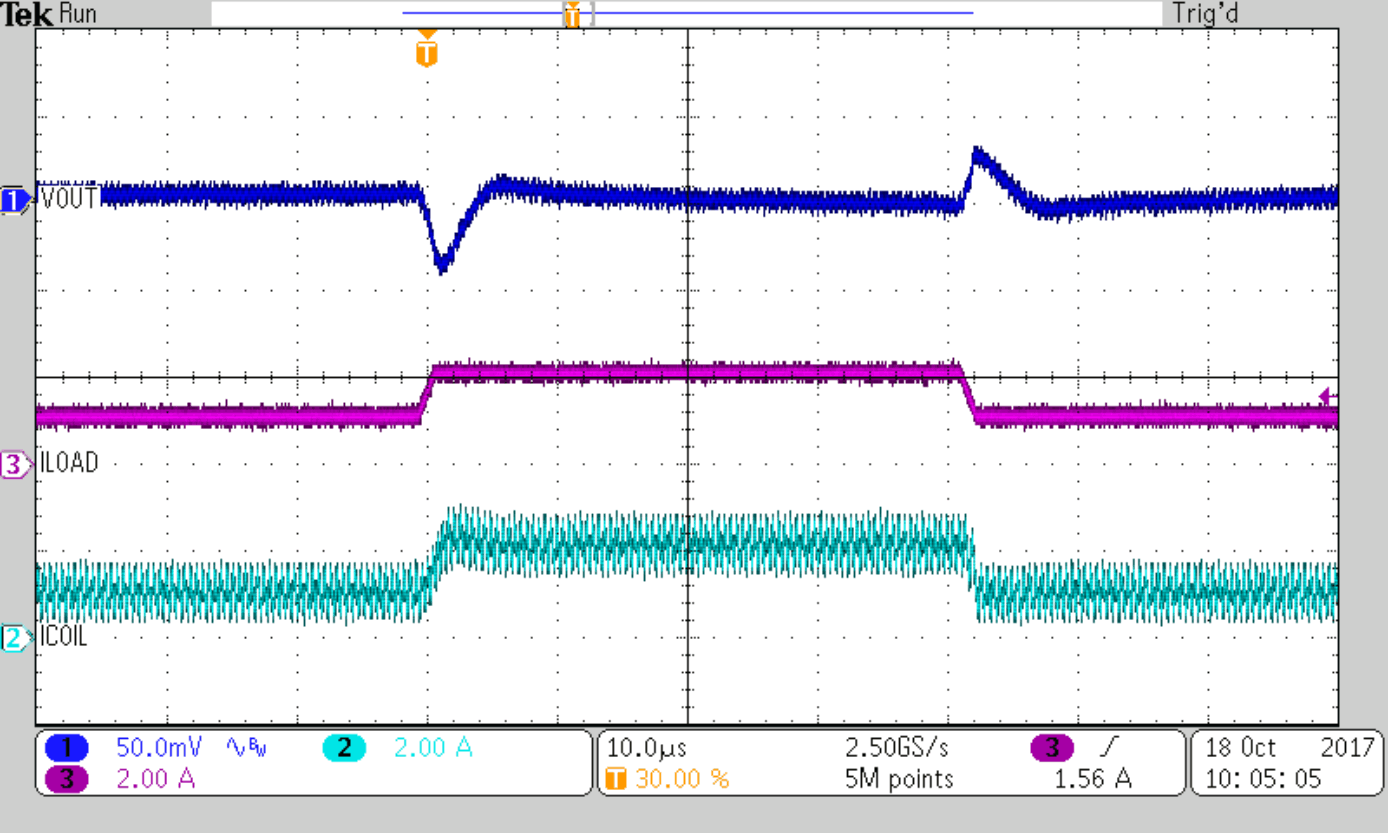COUT=2x10µF Cff=120pF
Figure 43. Load Transient Response, 1A to 2A, TPS62822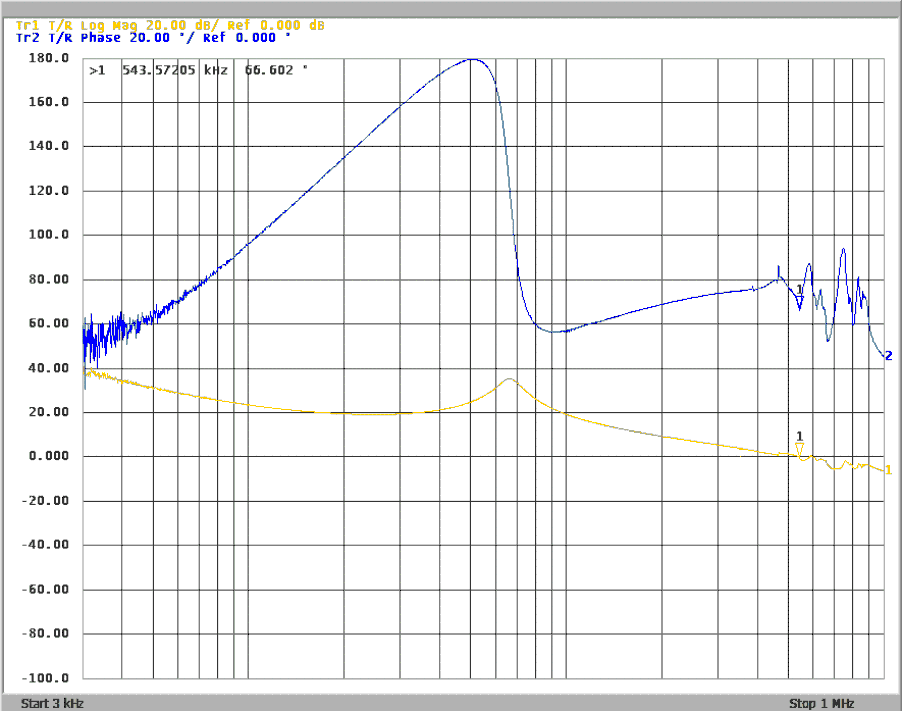COUT=2x10µF CFF=120pF
Figure 45. Frequency Response (TPS62823), IOUT=3A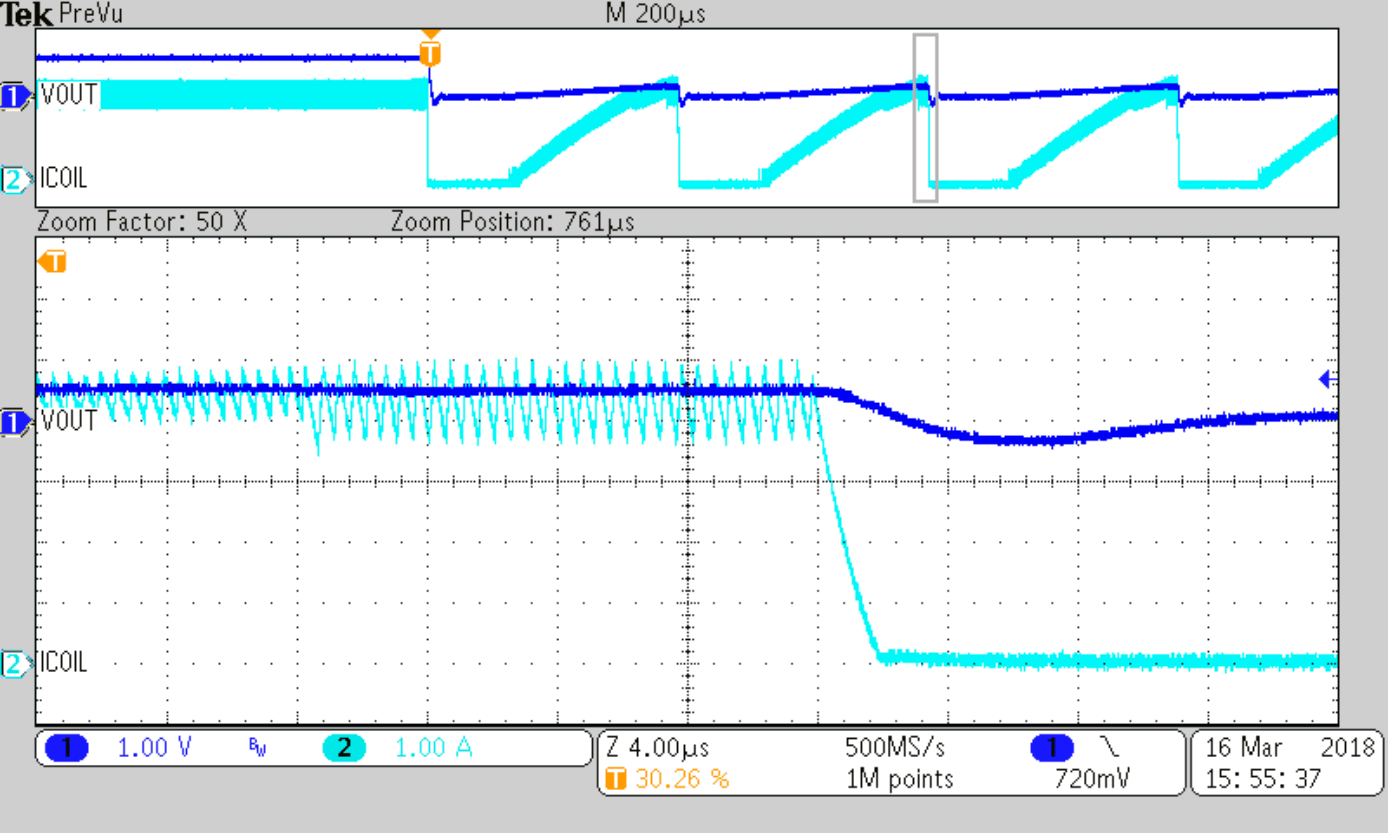Figure 47. Overload Response of TPS62823 (Hiccup cycle)